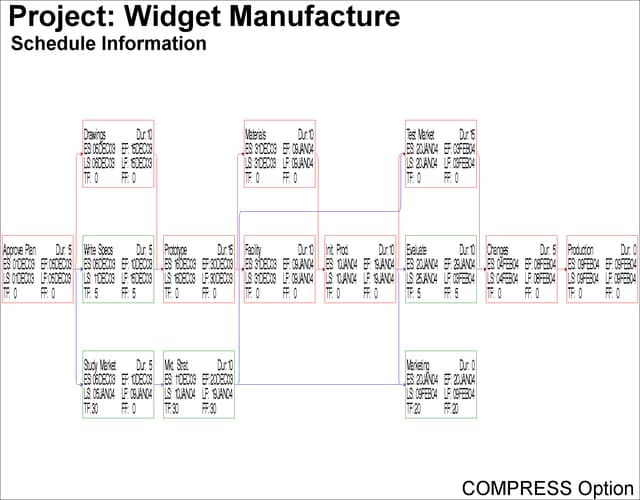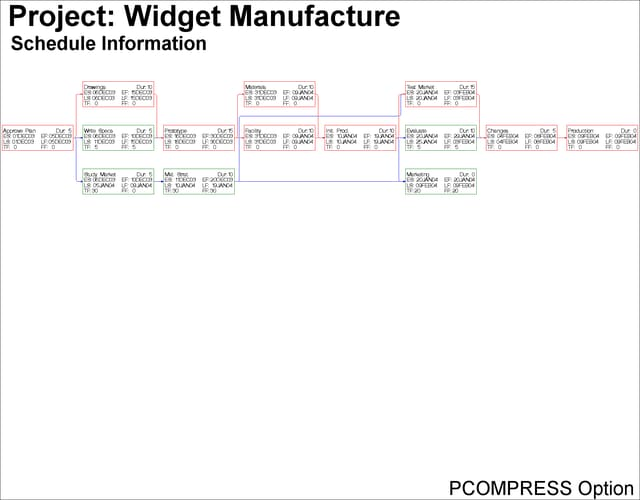### Example 9.4 The COMPRESS and PCOMPRESS Options

In Example 9.2, the number of character cell positions were specified so that the entire network fit on one page; in Example 9.3, the network spanned several pages. To force the network diagram to fit on one page automatically, you can use the COMPRESS or PCOMPRESS options. Both options use window transformations to fit the network on one page: the COMPRESS option treats the horizontal and vertical transformations independently of each other so that one direction may not be as compressed as the other; the PCOMPRESS option uses a proportional transformation so that each direction is compressed as much as the other. The following two invocations of PROC NETDRAW illustrate the differences in the diagrams produced.

In both network diagrams, PATTERN statements are used to color the nodes red or green, depending on whether the activities they represent are critical or not. The first PATTERN statement is for nodes corresponding to noncritical activities, and the second statement is for critical activities. The second invocation of PROC NETDRAW also uses the NOVCENTER option in the ACTNET statement to turn off centering in the vertical direction, so that the network is drawn immediately below the titles.

```goptions border;

pattern1 v=e c=green;
pattern2 v=e c=red;

title j=l h=3 ' Project: Widget Manufacture';
title2 j=l h=2 '  Schedule Information';
footnote j=r h=2 'COMPRESS Option ';
proc netdraw data=sched graphics;
succ=(succ1 succ2 succ3)
dur = days
carcs=blue
ccritarcs=red
separatearcs
font=swiss
height=1.5
compress;
run;
```
```footnote j=r h=2 'PCOMPRESS Option ';
proc netdraw data=sched graphics;
succ=(succ1 succ2 succ3)
dur = days
carcs=blue
ccritarcs=red
separatearcs
font=swiss
height=1.5
pcompress
novcenter;
run;
```

Output 9.4.1: Project Network: COMPRESS OptionOutput 9.4.2: Project Network: PCOMPRESS Option